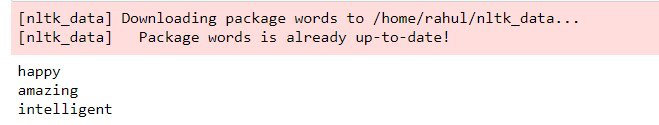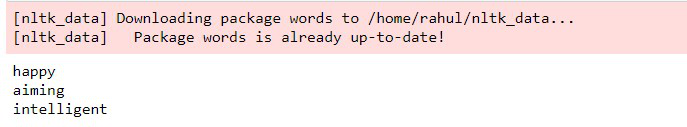Related Articles

# Correcting Words using NLTK in Python

• Last Updated : 18 Jul, 2021

nltk stands for Natural Language Toolkit and is a powerful suite consisting of libraries and programs that can be used for statistical natural language processing. The libraries can implement tokenization, classification, parsing, stemming, tagging, semantic reasoning, etc. This toolkit can make machines understand human language.

We are going to use two methods for spelling correction. Each method takes a list of misspelled words and gives the suggestion of the correct word for each incorrect word. It tries to find a word in the list of correct spellings that has the shortest distance and the same initial letter as the misspelled word. It then returns the word which matches the given criteria. The methods can be differentiated on the basis of the distance measure they use to find the closest word.  ‘words’ package from nltk is used as the dictionary of correct words.

### Method 1: Using Jaccard distance Method

Jaccard distance, the opposite of the Jaccard coefficient, is used to measure the dissimilarity between two sample sets. We get Jaccard distance by subtracting the Jaccard coefficient from 1. We can also get it by dividing the difference between the sizes of the union and the intersection of two sets by the size of the union. We work with Q-grams (these are equivalent to N-grams) which are referred to as characters instead of tokens. Jaccard Distance is given by the following formula.### Stepwise implementation

Step 1: First, we install and import the nltk suite and Jaccard distance metric that we discussed before. ‘ngrams’ are used to get a set of co-occurring words in a given window and are imported from nltk.utils package.

## Python3

 `# importing the nltk suite ``import` `nltk`` ` `# importing jaccard distance``# and ngrams from nltk.util``from` `nltk.metrics.distance ``import` `jaccard_distance``from` `nltk.util ``import` `ngrams`

Step 2: Now, we download the ‘words’ resource (which contains the list of correct spellings of words) from the nltk downloader and import it through nltk.corpus and assign it to correct_words.

## Python3

 `# Downloading and importing``# package 'words' from nltk corpus``nltk.download(``'words'``)``from` `nltk.corpus ``import` `words`` ` ` ` `correct_words ``=` `words.words()`

Step 3: We define the list of incorrect_words for which we need the correct spellings. Then we run a loop for each word in the incorrect words list in which we calculate the Jaccard distance of the incorrect word with each correct spelling word having the same initial letter in the form of bigrams of characters. We then sort them in ascending order so the shortest distance is on top and extract the word corresponding to it and print it.

## Python3

 `# list of incorrect spellings``# that need to be corrected ``incorrect_words``=``[``'happpy'``, ``'azmaing'``, ``'intelliengt'``]`` ` `# loop for finding correct spellings``# based on jaccard distance``# and printing the correct word``for` `word ``in` `incorrect_words:``    ``temp ``=` `[(jaccard_distance(``set``(ngrams(word, ``2``)),``                              ``set``(ngrams(w, ``2``))),w)``            ``for` `w ``in` `correct_words ``if` `w[``0``]``=``=``word[``0``]]``    ``print``(``sorted``(temp, key ``=` `lambda` `val:val[``0``])[``0``][``1``])`

Output:Output screenshot after implementing Jaccard Distance to find correct spelling words

### Method 2: Using Edit distance Method

Edit Distance measures dissimilarity between two strings by finding the minimum number of operations needed to transform one string into the other. The transformations that can be performed are:

• Inserting a new character:
`bat -> bats (insertion of 's')`
• Deleting an existing character.
`care -> car (deletion of 'e')`
• Substituting an existing character.
`bin -> bit (substitution of n with t)`
• Transposition of two existing consecutive characters.
`sing -> sign (transposition of ng to gn)`

### Stepwise implementation

Step 1: First of all, we install and import the nltk suite.

## Python3

 `# importing the nltk suite ``import` `nltk`` ` `# importing edit distance  ``from` `nltk.metrics.distance  ``import` `edit_distance`

Step 2: Now, we download the ‘words’ resource (which contains correct spellings of words) from the nltk downloader and import it through nltk.corpus and assign it to correct_words.

## Python3

 `# Downloading and importing package 'words'``nltk.download(``'words'``)``from` `nltk.corpus ``import` `words``correct_words ``=` `words.words()`

Step 3: We define the list of incorrect_words for which we need the correct spellings. Then we run a loop for each word in the incorrect words list in which we calculate the Edit distance of the incorrect word with each correct spelling word having the same initial letter. We then sort them in ascending order so the shortest distance is on top and extract the word corresponding to it and print it.

## Python3

 `# list of incorrect spellings``# that need to be corrected ``incorrect_words``=``[``'happpy'``, ``'azmaing'``, ``'intelliengt'``]`` ` `# loop for finding correct spellings``# based on edit distance and``# printing the correct words``for` `word ``in` `incorrect_words:``    ``temp ``=` `[(edit_distance(word, w),w) ``for` `w ``in` `correct_words ``if` `w[``0``]``=``=``word[``0``]]``    ``print``(``sorted``(temp, key ``=` `lambda` `val:val[``0``])[``0``][``1``])`

Output:Output screenshot after implementing Edit Distance to find correct spelling words

Attention geek! Strengthen your foundations with the Python Programming Foundation Course and learn the basics.

To begin with, your interview preparations Enhance your Data Structures concepts with the Python DS Course. And to begin with your Machine Learning Journey, join the Machine Learning – Basic Level Course

My Personal Notes arrow_drop_up